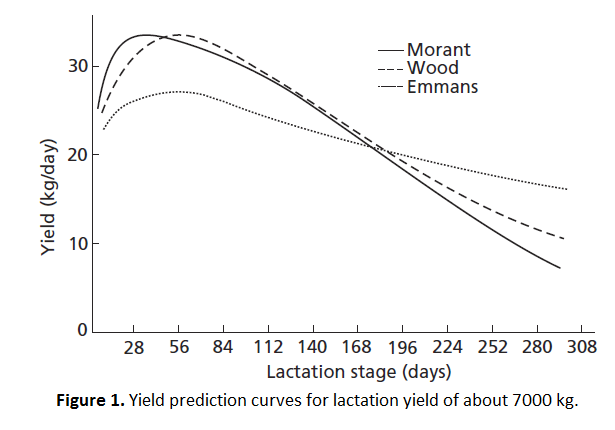top of page
Search
• P. McDonald, R. A. Edwards, J. F. D. Greenhalgh

# Milk Yield Of Dairy CowsThe yield of milk is mainly decided by the type of breed of the cow. For main British dairy breeds, the typical yield in descending order is shown in Table 1.

There are, however, considerable intrabreed variations with strain and individuality. Thus, it is possible for certain strains and individuals of low-yielding breed to outyield others of higher-yielding breed.

As a rule of thumb, older cows tend to have higher yield compared to younger cows. However, the main short term factor affecting milk yield is the stage of lactation. Yield in machine milked cows generally increases from parturition to about 35 days postpartum and then falls regularly at the rate of about 2–2.5 per cent per week(1.5–2.0 per cent for first-lactation animals) to the end of lactation. In individual cases, yield frequently reaches a peak earlier in lactation and the fall thereafter is much sharper.

As a result of several factors affecting yield, the yield of milk may vary over a very wide range. Fortunately, such variations present little difficulty in assessing the nutrients required for the dairy cow since yield is easily and conveniently measured.

For long-term planning of the feeding of the lactation cow, estimates of yield are necessary. Therefore, several useful generalisations may be made in order to allow prediction of yield at a given stage of lactation.

Peak yield may be calculated as one two-hundredth of the expected lactation yield (one two-hundred and fiftieth for first-lactation animals) or as 1.1 times the yield recorded 2 weeks postpartum, e.g. a fourth-lactation cow yielding 27 kg at this time could be expected to have a peak yield of 30 kg and have a lactation yield of 6000 kg.The assumption of a weekly rate of decline from peak yield of 2.5 per cent per week is useful in predicting milk yield and also in monitoring deviations from normality during the progress of lactation.Such estimates are relatively imprecise, and attempts to increase accuracy have resulted in highly sophisticated mathematical descriptions of the changes in yield with lactation.

In 1976,Wood (see Further reading) suggested that the yield of milk on any day postpartum (y) may be calculated using equations of the following type:where n = week of location, a = a positive scalar directly related to total milk production, b = an index of the animal’s capacity to utilise energy for milk production, and c = a decay rate.

Values for a, b and c may be obtained from lactation yield data by a least-squares procedure. The constants for yield groups may then be used to predict week of peak yield (b/c) and daily yield in a given week of lactation.Typical figures for a cow with an expected lactation yield of 5500 kg would be: a = 26.69 b = 0.03996 c = 0.00942

Week of peak yield would then be 4.24 (0.03996/0.00942) and predicted maximum daily yield 27.17 kg. The Wood equation has been criticised in that it predicts zero yield at parturition, which, in the light of the development of the secretory potential of the mammary gland at parturition, is obviously not so. A number of alternatives have been put forward. Most have been more complex and have proved to give less satisfactory performance. That of Emmans and Fisher 1986marginally outperforms the Wood equation, the proportion of variation explained (R²) being 0.84 compared with 0.82, and dispersion around the regression line (residual standard deviation) being 1.74 compared with 1.86. In addition, it does not predict zero yield at parturition and is more easily interpretable in biological terms. It does so, however, at the expense of increased complexity and the introduction of an extra term.

The Agricultural and Food Research Council in 1993 proposed the use of the Morant equation (1989):where b, c and k  constants varying with parity and lactation yield, a = the natural log of the expected yield at day 150 of lactation, t = days since calving, and tl = (t - 150)100.Figure 1. shows constructed yield curves for the lactation of a cow yielding about 7000 kg of milk in 48 weeks.

*Article reposted based on Animal Nutrition 7th edition